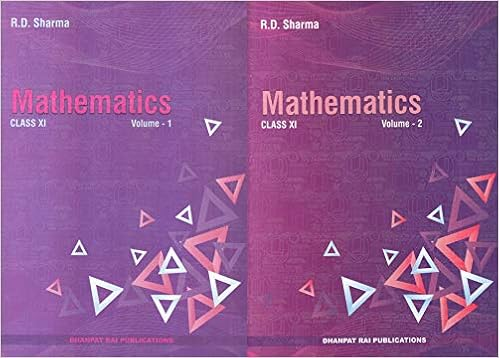# [PDF] RD Sharma Mathematics for Class 11 (set of 2 volumes) Examination | Download

About The R D Sharma Class 11 Maths Book
This textbook is based on the latest syllabus prescribed by the CBSE. The text has been divided into two volumes. Volume 1 Consists of chapters 1-21 and Volume 2 Consists of chapters 22-23 for ease of handling. Illustrative examples and exercises are given at the end of every section in each chapter at the end of each chapter exercises consisting of MCQs, Fill in the blanks, Very Short Answer Type questions, and activities have been given. Summary for quick revision concepts and formulae have also been given. Ncert problems in the exercises have been solved in the section "hints to NCERT & selected problems".Salient Features

The Mathematics for Class 11 by R D Sharma (Set of 2 Volume) closely follows the CBSE syllabus for Mathematics and is suitable for the students to develop their Mathematical skills. It contains detailed solutions to Mathematical equations and problems which are further simplified by the representation of elaborate yet simple to understand steps. It covers all the genres or branches of Mathematics which will further act as building blocks for the Mathematical knowledge based on the students of class 11. This book will be of great help if one is looking to crack entrance examinations that are offered by prestigious academies for courses in which one can enroll themselves after their class 11 board examinations.

RD Sharma Mathematics for Class 11 (set of 2 volumes) PDF
Volume 1
Volume 2
Other Free Materials
1.